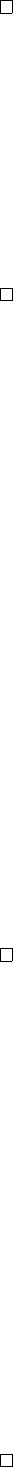8Matrix groups

IA Groups8.4 Rotations and reflections in R
2
and R
3
Lemma. SO(2) consists of all rotations of R
2
around 0.
Proof.
Let
A SO
(2). So
A
T
A
=
I
and
det A
= 1. Suppose
A
=
a b
c d
.
Then
A
1
=
d b
c a
.
So
A
T
=
A
1
implies
= 1,
c
=
b
,
d
=
a
.
Combining these equations we obtain
a
2
+
c
2
= 1. Set
a
=
cos θ
=
d
, and
c = sin θ = b. Then these satisfies all three equations. So
A =
cos θ sin θ
sin θ cos θ
.
Note that
A
maps (1
,
0) to (
cos θ, sin θ
), and maps (0
,
1) = (
sin θ, cos θ
), which
are rotations by θ counterclockwise. So A represents a rotation by θ.
Corollary.
Any matrix in O(2) is either a rotation around 0 or a reflection in a
line through 0.
Proof. If A SO(2), we’ve show that it is a rotation. Otherwise,
A =
1 0
0 1
cos θ sin θ
sin θ cos θ
=
cos θ sin θ
sin θ cos θ
since O(2) =
SO
(2)
1 0
0 1
SO
(2). This has eigenvalues 1
,
1. So it is a
reflection in the line of the eigenspace
E
1
. The line goes through
0
since the
eigenspace is a subspace which must include 0.
Lemma. Every matrix in SO(3) is a rotation around some axis.
Proof.
Let
A SO
(3). We know that
det A
= 1 and
A
is an isometry. The
eigenvalues
λ
must have
|λ|
= 1. They also multiply to
det A
= 1. Since we are
in
R
, complex eigenvalues come in complex conjugate pairs. If there are complex
eigenvalues
λ
and
¯
λ
, then
λ
¯
λ
=
|λ|
2
= 1. The third eigenvalue must be real and
has to be +1.
If all eigenvalues are real. Then eigenvalues are either 1 or
1, and must
multiply to 1. The possibilities are 1
,
1
,
1 and
1
,
1
,
1, all of which contain an
eigenvalue of 1.
So pick an eigenvector for our eigenvalue 1 as the third basis vector. Then in
some orthonormal basis,
A =
a b 0
c d 0
0 0 1
Since the third column is the image of the third basis vector, and by orthogonality
the third row is 0, 0, 1. Now let
A
0
=
a b
c d
GL
2
(R)
with
det A
0
= 1.
A
0
is still orthogonal, so
A
0
SO
(2). Therefore
A
0
is a rotation
and
A =
cos θ sin θ 0
sin θ cos θ 0
0 0 1
in some basis, and this is exactly the rotation through an axis.
Lemma.
Every matrix in O(3) is the product of at most three reflections in
planes through 0.
Note that a rotation is a product of two reflections. This lemma effectively
states that every matrix in O(3) is a reflection, a rotation or a product of a
reflection and a rotation.
Proof.
Recall O(3) =
SO
(3)
1 0 0
0 1 0
0 0 1
SO
(3). So if
A SO
(3), we know
that
A
=
cos θ sin θ 0
sin θ cos θ 0
0 0 1
in some basis, which is a composite of two
reflections:
A =
1 0 0
0 1 0
0 0 1
cos θ sin θ 0
sin θ cos θ 0
0 0 1
,
Then if
A
1 0 0
0 1 0
0 0 1
SO
(3), then it is automatically a product of three
reflections.
In the last line we’ve shown that everything in O(3)
\ SO
(3) can be written as
a product of three reflections, but it is possible that they need only 1 reflection.
However, some matrices do genuinely need 3 reflections, e.g.
1 0 0
0 1 0
0 0 1